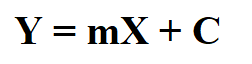# Summary Notes for Linear Law – O Level Add MathLinear law is a chapter that revolves around making a non-linear relationship linear by changing our concept of y must be the vertical axis and x must be the horizontal axis. I talked about what is tested for linear law in O Level Additional Mathematics in my previous post, which you can read about here.

In this post, let’s do a quick summary on what you need to know for linear law for O Level Add Math.

## Concepts from Linear Law

The entire of this topic revolves making an equation in the form Y = mX +C where Y and X are variables. Y  and X just needs to be variables. They need not be y or x, but can be log y, x/y or anything. m is the gradient of the graph of Y against X, and C is the vertical intercept. Both m and C must be constant terms (which are usually unknown), and cannot have any variable terms in them.

## Example of application of Linear Law

Let’s assume that we have the variables y and x that follow this equation y = axn, where a and n are constants to be determined. Now, we are given a set of values of x and y.

How would you determine the values of a and n using concepts learnt in straight line graph?

If we were to plot y against x, base on observation we’ll definitely get a curve (meaning relationship between x and y is not linear). So, what happens when we apply natural logarithm (or ln) to both sides of the equation?

ln both sides of : y = axn

Apply ln to both sides of the equation, the equation is transformed to this: lny = nlnx + lna

Based on the general formula of straight line graph, Y = mX + C, if we were to plot lny against lnx, then n will be the gradient and lna will be the vertical intercept.

Now, I’ve set up a table with lnx and lny in it.

Let’s plot these points. The graph of lny against lnx is as shown below:

Once we have plot the graph, next is to find the vertical intercept (which is about 0.69 from the graph). Since we know that the vertical intercept is equal to lna,  a= e 0.69 which is about 2.

Next we’ll find the gradient (by making use of 2 points on the straight line). The gradient of the graph is equal to n. If you were to work it out, n should be about 3.5.

## Want to learn O Level Additional Mathematics on-demand?

Mathematics is all about applying and being able to do questions. Apart from knowing these notes and formulae, you must also be familiar with how to apply them to the questions. The nice thing about Additional Mathematics is the questions are quite standard.

For instance, there are generally 2 main types of questions for linear law tested in O Level Additional Mathematics.

In my course, I go into detail not only the concepts but how to apply them. We also go through many questions together step-by-step, so that students finish the course equipped with the skills needed to tackle their test and exam questions with confidence. You can get the course here.

Want to sample the course? Watch our sample video on a typical question on linear law here.

error: Content is protected !!